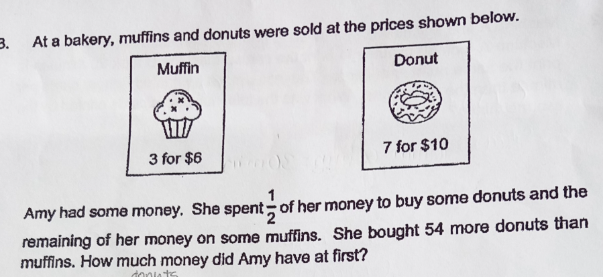# QuestionThank you!

This question is easier to use algebra to find the factors for each type with a difference of 54.

Let d be the factor for donuts and m be the factor for muffins.

7d – 3m = 54    (donuts bought was 54 more than muffins)

6m = 10d          (same amount was spent on both since she spent 1/2 her money on donuts =>

half was on muffins)

m = (10/6)d = (5/3)d

7d – 3(5/3)d = 54

2d=54 => d = 27

m = (5/3) x 27 = 5 x 9 = 45

Hence total amount of money Amy has at first is 6m +10d = 270 + 270 = \$540

0 Replies 1 Like